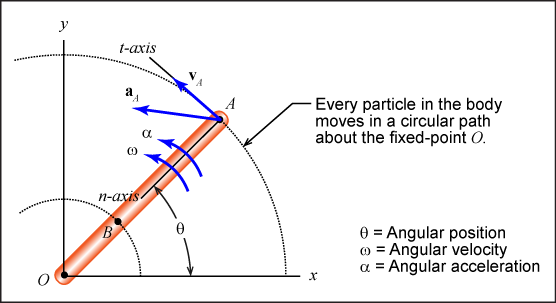# Pure Rotation

### Rotation about a fixed axis

Pure rotation occurs when a body rotates about a fixed non-moving axis. The following figure illustrates fixed-axis rotation.### Motion characteristics

• Angular position: (θ) Angular position relative to an inertial coordinate axis. Similar to how θ was measured in polar coordinates.
• Angular velocity: (ω) How fast a body is rotating.
• Angular acceleration: (α) How fast a body's rotation rate is increasing or decreasing.

### Angular kinematic relationships

• Angular velocity: The time rate of change of angular position. ω = dθ/dt
• Angular acceleration: The time rate of change of angular acceleration. α = dω/dt = d 2θ/dt 2
• Angular velocity / Angular acceleration: Combining the angular velocity and acceleration equations, we obtain α dθ = ω dω.

### Angular motion parameter units

#### What are the units for angular position?

Check all that apply.

#### What are the units for angular velocity?

Check all that apply.

#### What are the units for angular acceleration?

Check all that apply.